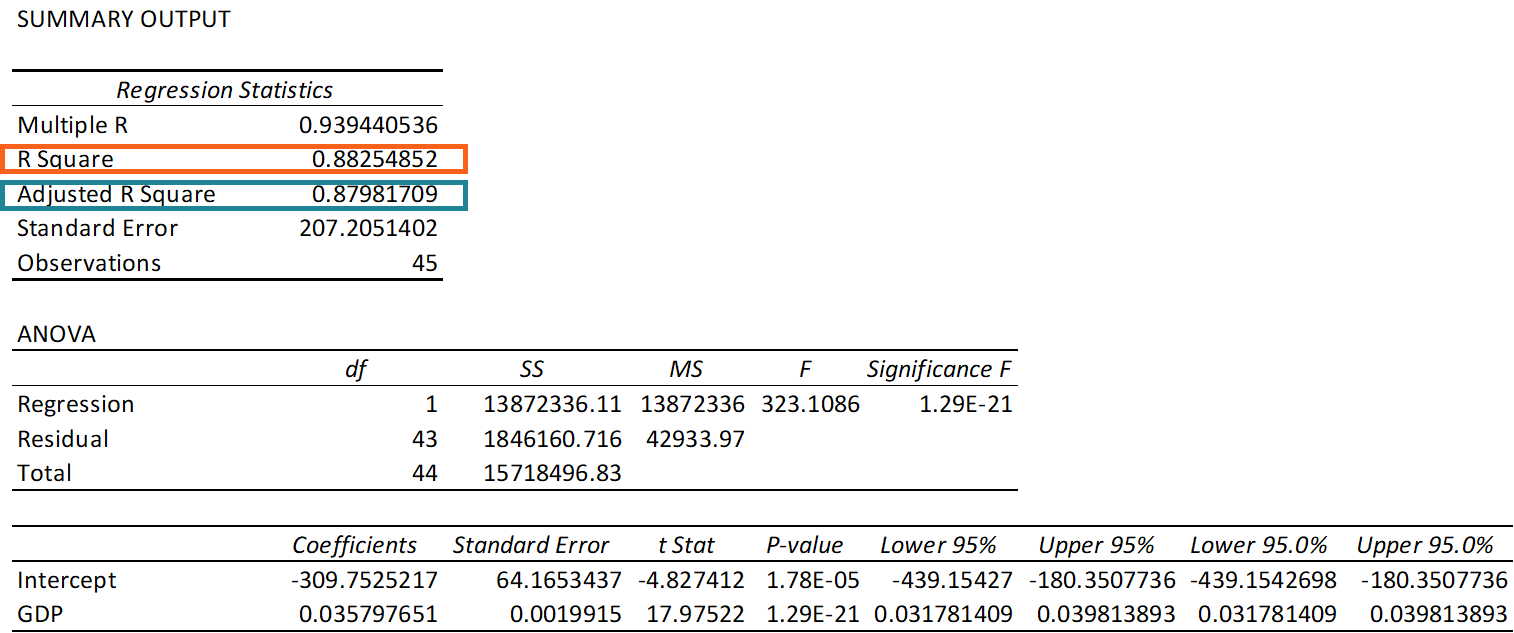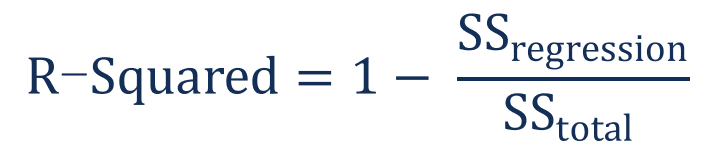# R-Squared

A statistical measure that determines the proportion of variance in the dependent variable that can be explained by the independent variable

## What is R-Squared?

R-Squared (R² or the coefficient of determination) is a statistical measure in a regression model that determines the proportion of variance in the dependent variable that can be explained by the independent variable. In other words, r-squared tells how well the data fit the regression model (the goodness of fit).Figure 1. Regression output in MS Excel

R-squared can take any values between 0 to 1. Although the statistical measure provides some useful insights regarding the regression model, the user should not rely only on the measure in the assessment of a statistical model. The figure does not disclose information about the causation relationship between the independent and dependent variables.

In addition, it does not indicate the correctness of the regression model. Therefore, the user should always draw conclusions about the model by analyzing r-squared together with the other variables in a statistical model.

### Interpretation of R-Squared

The most common interpretation of r-squared is how well the regression model fits the observed data. For example, an r-squared of 60% reveals that 60% of the data fit the regression model. Generally, a higher r-squared indicates a better fit for the model.

However, it is not always the case that a high r-squared is good for the regression model. The quality of the statistical measure depends on many factors, such as the nature of the variables employed in the model, the units of measure of the variables, and the applied data transformation. Thus, sometimes, a high r-squared can indicate the problems with the regression model.

A low r-squared figure is generally a bad sign for predictive models. However, in some cases, a good model may show a small value.

There is no universal rule on how to incorporate the statistical measure in assessing a model. The context of the experiment or forecast is extremely important and in different scenarios, the insights from the metric can vary.

### How to Calculate R-Squared

The formula for calculating R-squared is:Where:

• SSregression is the sum of squares due to regression (explained sum of squares)
• SStotal is the total sum of squares

Although the names “sum of squares due to regression” and “total sum of squares” seem confusing, the meanings of the variables are straightforward.

The sum of squares due to regression measures how well the regression model represents the data that were used for modeling. The total sum of squares measures the variation in the observed data (data used in regression modeling).